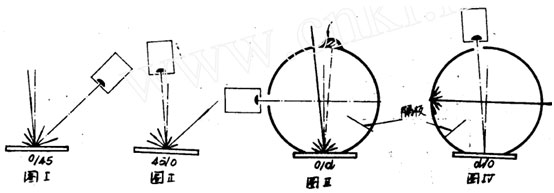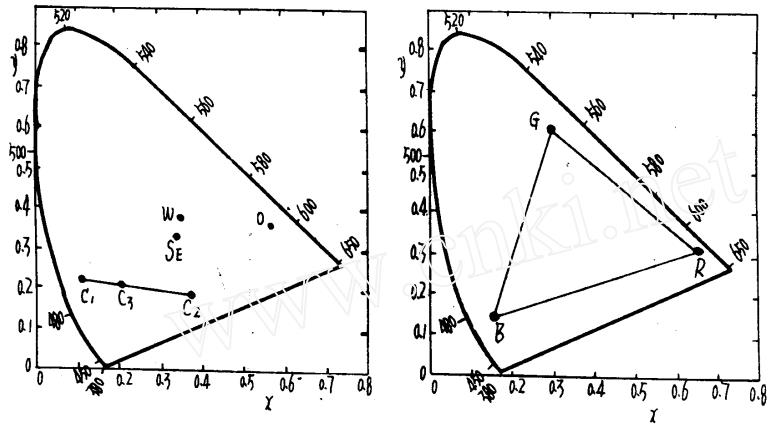色差仪照明观测的几何条件X=100XL/YLP
Y=100YL/YLP=100L,L
Z=100ZL/YLP

X=X/(X+Y+Z)
Y=Y/(X+Y+Z)
Z=Z/(X+Y+Z)
X+Y+Z=1

X，Y，Y，L
X，Y，L
X，Y色度图的特性SE•X=1/3 Y=1/3

Pi5红包